# Javascript 变量生命周期

ES6 为`let`提供了一个不同的和改进的机制。它要求更严格的变量声明,在定义之前不能使用，从而提高代码质量。

## 1. 容易出错的 var 提升

``````// var hoisting
num;     // => undefined
var num;
num = 10;
num;     // => 10
// function hoisting
getPi;   // => function getPi() {...}
getPi(); // => 3.14
function getPi() {
return 3.14;
}

## 2. 理解背后原理：变量生命周期

1. **声明阶段(Declaration phase)**是在作用域中注册一个变量。

2. **初始化阶段(Initialization phase)**是分配内存并为作用域中的变量创建绑定。 在此步骤中，变量将使用`undefined`自动初始化。

3. **赋值阶段(Assignment phase)**是为初始化的变量赋值。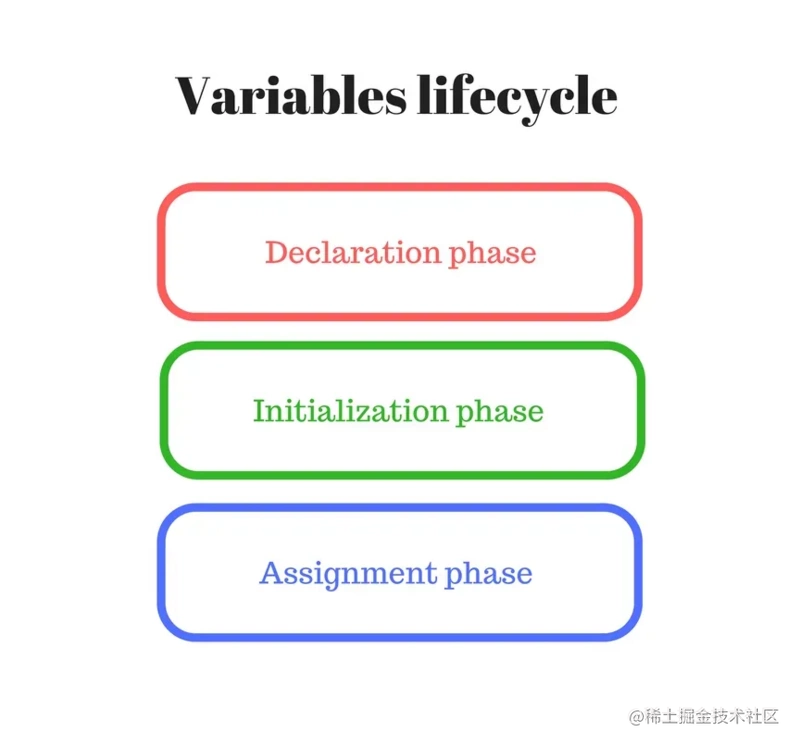## 3.var 变量的生命周期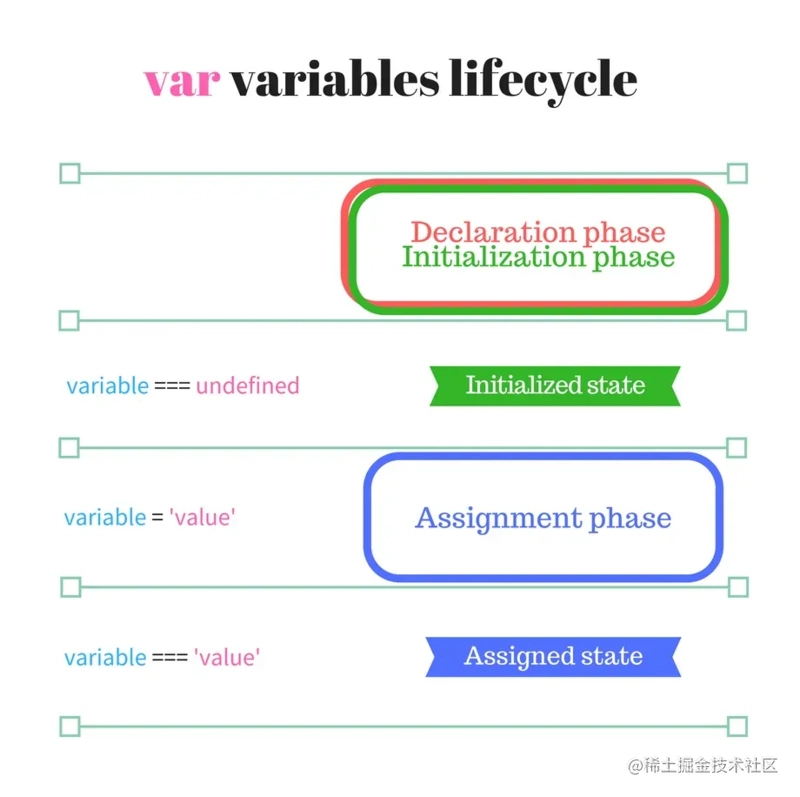``````function multiplyByTen(number) {
console.log(ten); // => undefined
var ten;
ten = 10;
console.log(ten); // => 10
return number * ten;
}
multiplyByTen(4); // => 40

## 4. 函数声明生命周期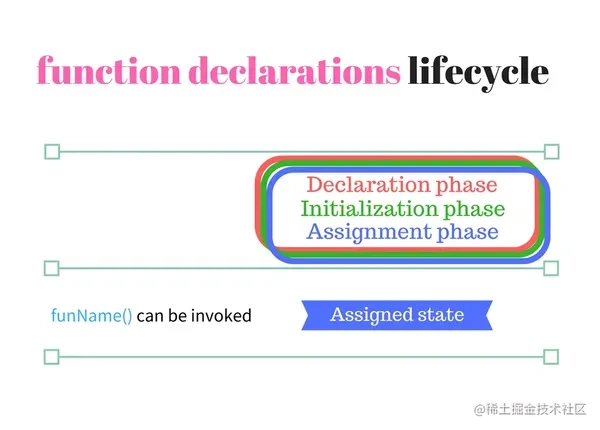``````function sumArray(array) {
return array.reduce(sum);
function sum(a, b) {
return a + b;
}
}
sumArray([5, 10, 8]); // => 23

## 5. let 变量的生命周期

`let` 变量的处理方式与`var`不同，主要区别在于声明和初始化阶段是分开的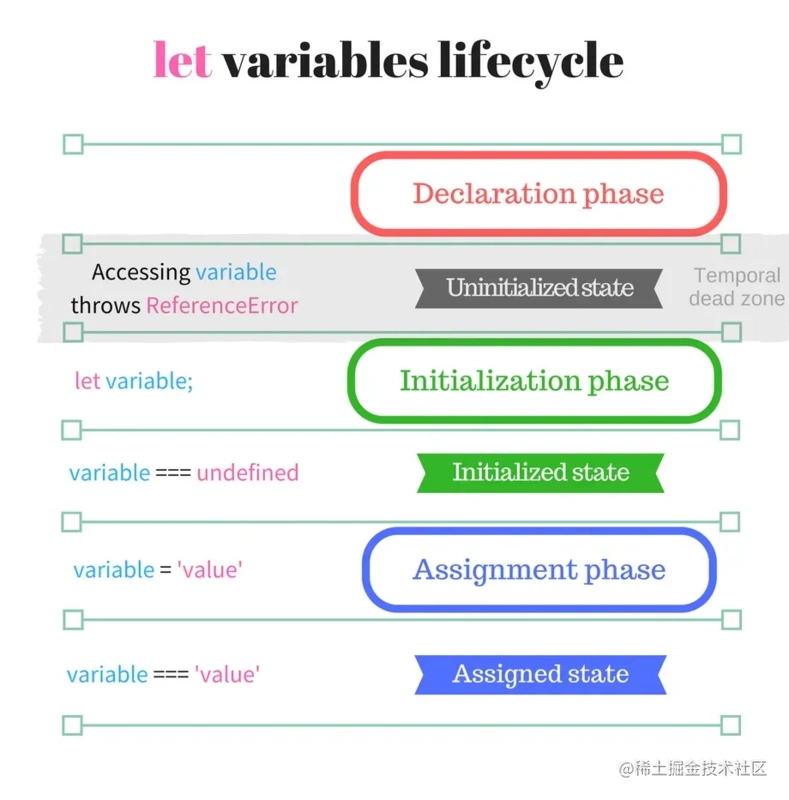``````let condition = true;
if (condition) {
// console.log(number); // => Throws ReferenceError
let number;
console.log(number); // => undefined
number = 5;
console.log(number); // => 5
}

`const``class` 类型与`let`具有相同的生命周期，只是分配只能发生一次。

## 总结

• 声明、初始化然后使用变量，这个流程是正确的，易于遵循。

• 尽量隐藏变量。公开的变量越少，代码就越模块化。

## 番外

#### 如何理解 let x = x 报错之后，再次 let x 依然会报错？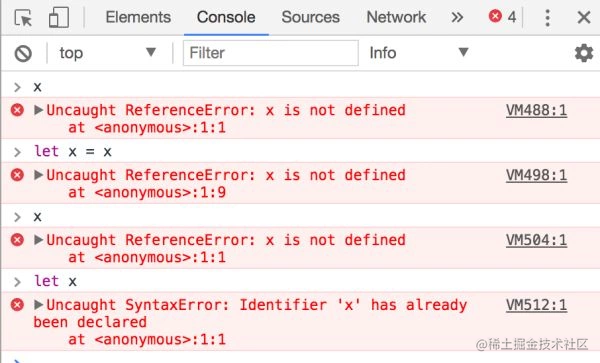1. x 变量就将永远处于 created 状态。

2. 你无法再次对 x 进行初始化（初始化只有一次机会，而那次机会你失败了）。

3. 由于 x 无法被初始化，所以 x 永远处在暂时死区

4. 有人会觉得 JS 坑，怎么能出现这种情况；其实问题不大，因为此时代码已经报错了，后面的代码想执行也没机会。